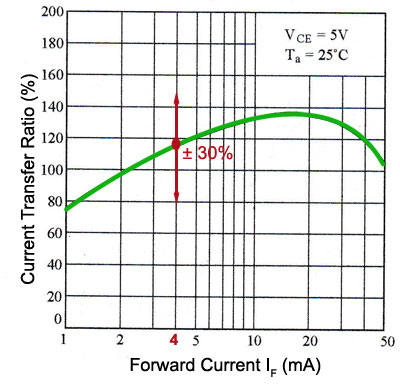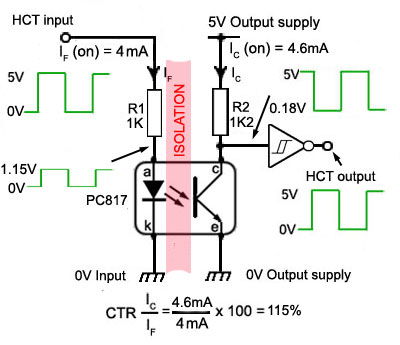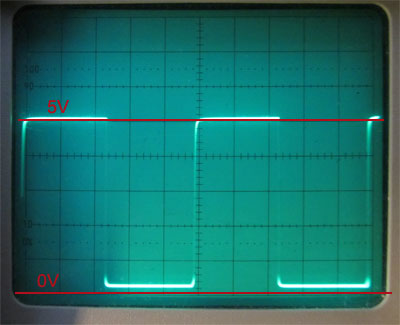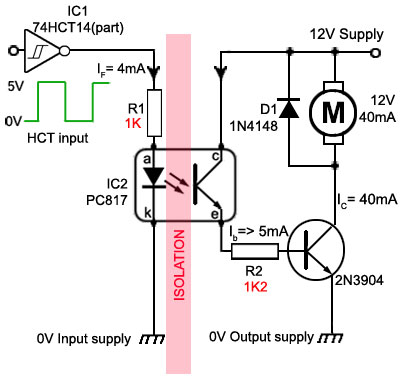# Using Optocouplers

• After studying this section, you should be able to:
• Describe basic applications of optocouplers:
• Understand the design of optocoupler circuits
• • Using the Current Transfer Ratio (CTR) .
• • Calculating component values for optocouplers.
• Understand the requirements for a typical optocoupler application.
• • Level shifting.
• • Input/output isolation.
• • Driving high current loads.
• • Back emf protection.

There are many different applications for optocoupler circuits, so there are many different design requirements, but a basic design for an optocoupler providing isolation for example between two circuits, simply involves the choice of appropriate resistor values for the two resistors R1 and R2 shown in Fig. 5.2.1.

In this example a PC817 optocoupler is shown isolating a circuit using HCT logic via a 7414 Schmitt inverter gate. The Schmitt inverter at the output performs several functions; it ensures that the output conforms to HCT voltage and current specifications, it also provides very fast rise and fall times for the output, and corrects the signal inversion caused by the phototransistor being operated in common emitter mode. Each logic family (e.g. LSTTL or CMOS types) may have different logic voltage levels and different input and output current requirements, and optocouplers can provide a convenient way of interfacing two circuits with different logic levels. What is necessary is to ensure that R1 creates an appropriate current level from the input circuit to correctly drive the LED side of the optocoupler, and that R2 creates appropriate voltage and current levels to supply the output circuit via the inverter.## Designing Optocoupler Interfaces

The main purpose of an optocoupler interface is to completely isolate the input circuit from the output circuit, which normally means there will be two completely separate power supplies, one for the input circuit and one for the output. In this simple example the input and output supplies will most likely be the same in voltage and current capabilities, so the interface is just providing isolation without any major shift in voltage or current levels.

In choosing appropriate values for R1, the value for the current limiting resistor is set to produce the correct forward current (IF) through the infrared LED in the optocoupler. R2 is the load resistor for the phototransistor and the values of both resistors will depend on a number of factors.

## Current Transfer Ratio

The current in each half of the circuit is linked by the Current Transfer Ratio or CTR, which is simply the ratio of output current to the input current (IC/IF) usually expressed as a percentage. Each optocoupler type will have a range of CTR values set out in the manufacturer's datasheet. The value of CTR also depends on a number of factors, first of all is the type of optocoupler, simple types may have a CTR value of between 20% and 100%, whilst special types, such as those that use a Darlington transistor configuration for their output phototransistor, may have CTR values of several hundred percent. Also the CTR of any particular device may vary considerably from that device's typical value by anything up to +/-30%. Manufacturers will normally quote a range of CTR values for different output phototransistor collector voltages (VC) and different ambient temperatures (TA) The CTR will also vary with the age of the optocoupler, as the efficiency of LEDs decreases with age (over 1000s of operating hours). Because the CTR of an optocoupler can be expected to reduce over time, it is common practice to choose a value for IF somewhat lower than the maximum, so that the intended performance can still be achieved over the intended lifetime of the circuit.

Although this example describes the design of a simple interface linking two HCT logic circuits, the difference between the results achieved here and those needed for any other optocoupler are that similar calculations can be made just using data appropriate to other voltages and currents and other optocouplers.

## Calculating the Optocoupler Resistor Values### Fig. 5.2.2 CTR vs. Forward Current for a PC817

The start of the design process is to specify the input and output conditions the optocoupler is to link. Typical optocouplers can handle input and output currents from a few microamps to tens of milliamps. There are many optocouplers on the market and to find the most appropriate for a particular purpose, vendor's catalogues and manufacturers datasheets should be studied.

In this case however, a popular PC817 optocoupler from Sharp will use voltages and currents available from HCT logic. Assuming that a single HCT output is only feeding this optocoupler, a logic 1 voltage of about 4.9V can be presumed.

The output current available from a HCT gate to drive the optocoupler input is limited to 4mA, which is quite low for driving an optocoupler. The PC817 must then be capable of producing the necessary output from this low input current.

The graph in Fig. 5.2.2 shows, the CTR for a PC817 with a forward (input) current IF of 4mA will be around about 80 to 150% allowing ±30% for all the variables mentioned above). Ideally the optocoupler should in this case act as though it is invisible, that is the HCT gate connected to the optocoupler output should see an available current of up to 4mA, just as though it was connected to the output of another HCT gate. Therefore the output current of the PC817 needs also to be ideally about 4mA, with the forward current (IF) driving the input LED at 4mA (assuming a 100% CTR).

Having found an approximate figure for the CTR, which suggests that input and output conditions should be similar, at 4mA, the next task is to calculate the values of R1 and R2.Using the data in Table 5.2.1 and assuming minimum 4.9V to 5V input at the HCT gate output, it is possible to calculate a suitable resistance value for R1 in Fig. 5.2.3.### Fig. 5.2.3 HCT to HCT Optocoupler

The forward Voltage across the infrared LED with a forward current of only 4mA should be about 1.2V

5V − 1.2V = 3.8V to be developed across R1

Therefore R1 = 3.8V÷4mA = 950Ω

Using the next higher preferred resistor value R1 = 1KΩ

The graph of CTR vs. IF in Fig. 5.2.2 shows that ideally the CTR for the PC817 will be about 115% with a forward current of 4mA, which suggests that the opto output current should be about 4mA x 115% = 4.6mA

To saturate the phototransistor and produce a logic 0 (less than 0.2V) at the output, R2 must develop a voltage of 4.9 to 5V when passing a current of 4.6mA (assuming 115% CTR value).

R2 must therefore be at least 5V÷4.6mA = 1087Ω or R2 = 1.2kΩ (next preferred value).### Fig. 5.2.4a Output with R2 = 1.2KΩ

If a higher value than 1.2KΩ is used, increasing this value by a few kΩ could ensure that the output has the maximum voltage swing, however increasing this value reduces the speed with which the optocoupler can can respond to fast voltage changes, due to the combination of a high resistance load and a high junction capacitance of the phototransistor, which results in a rounding of the output waveform, as can be seen by comparing the waveforms in Fig. 5.2.4 a & b.

Both of the waveforms shown were taken with the same input, a square wave with a frequency of 2kHz, but with two different values for R2, 1.2kΩ in Fig. 5.2.4a and 10kΩ in Fig. 5.2.4b.

The rounding effect on the rise time of the pulses can be clearly seen in Fig. 5.2.4b. Also at higher frequencies amplitude of the output signal reduces markedly. Therefore for best performance the value of R2 should be kept as low as possible, but above 1kΩ.### Fig. 5.2.4b Output with R2 = 10KΩ

The performance of the optocoupler circuit showing the result of using the calculated values is shown in Fig. 5.2.4. Note also the effect of using a 74HCT14 Schmitt inverter at the output; any rounding of the square pulses is eliminated and although the optocoupler output only falls to 0.18V when the phototransistor saturates, the output of the Schmitt gate actually changes between +5V and 0v.

Adding a Schmitt inverter also re-inverts the output waveform, which was an inverted version of the input waveform at the collector of the phototransistor.

There are of course, more useful applications for an optocoupler than simply isolating one logic IC from another. A common problem is driving a load from a computer's output port. Computers are expensive and easily damaged by mistakes made when connecting them to external circuitry. The problem is lessened by ensuring that the external circuit is fully isolated from the computer and an optocoupler such as the PC817 is a cheap and effective (assuming no major user errors) solution.## PC817 Motor Drive Circuit

Fig. 5.2.6 illustrates a typical example where it is required to drive a 12V DC motor requiring 40mA of current from a logic circuit (or typical computer port) that can only support a few mA of current at 5V or less.

As the current available from typical computer input/output ports may only be a few µA, as computer port lines are usually designed to drive some type of logic input, the input to this motor drive circuit is via a HCT Schmitt inverter gate, which only requires an input current of 1µA, with the 12V 40mA motor being driven by a 2N3904 transistor. The optocoupler infrared LED is driven at about 4mA via a 1kΩ resistor from IC1 output. As the CTR of the PC817 is around 115% the phototransistor can supply about 9mA as the supply to the phototransistor output is now taken from the 12V motor supply. This is more than the 5mA minimum required to drive the 2N3904 into saturation. It is important that the transistor is fully saturated in order to reduce the power dissipation in the 2N3904 to a minimum, therefore although the transistor current (ICE) is 40mA there will only be about 0.3V across the saturated transistor, so the power dissipation in the transistor will be 0.3V x 40mA = 12mW and the maximum dissipation for the 2N3904 is 1.5W. Although this basic interface only allows for switching the motor on or off, it could easily be adapted by changing IC1 to include a pulse width modulated speed control either from a computer, or hardware generated as described in Oscillators Module 4.6.

This simple interface has one more safety feature; diode D1 connected across the motor will effectively prevent any nasty back EMF spikes generated by the inductive load (the motor) from causing damage to the interface.

Top of Page